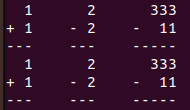# Arithmetic_arranger Help needed!

Tell us what’s happening:
When i run the tests the formatting looks correct with all the spaces and everything, however i still keeps getting 6 failed tests. It would be amazing if anyone can check out my code and help out thanks!

Your code so far

``````def arithmetic_arranger(problems, solve = False):
#final solution strings
firstline = ""
secondline = ""
lines = ""
sumdif = ""
strings = ""
if (len(problems) >= 6):
return "Error: Too many problems."
for problem in problems:
first_number = problem.split(" ")
operator = problem.split(" ")
second_number = problem.split(" ")
#operator can only be + or -
if (operator == "/" or operator == "*"):
return "Error: Operator must be '+' or '-'."
#numbers can only be a digit
if (first_number.isdigit() == False or second_number.isdigit() == False):
return "Error: Numbers must only contain digits."
#max of 4 digit width
if (len(first_number) >= 5 or len(second_number) >= 5):
return "Error: Numbers cannot be more than four digits."
# if solve is true solutions
if (operator == "+"):
answer += str(int(first_number) + int(second_number))
elif (operator == "-"):
answer += str(int(first_number) - int(second_number))
#find the length of the longer number
line = "-"
long_length = max((len(first_number)), len(second_number)) + 1
for d in range(long_length):
line += "-"
#returning/printing final values
if problems != problem[-1]:
firstline += first_number.rjust(len(line)) + "    "
secondline += operator+ " " + second_number.rjust(len(line)-2) + "    "
lines += line + "    "
sumdif += answer.rjust(len(line)) + "    "
else:
firstline += first_number.rjust(len(line))
secondline += operator + " " +second_number.rjust(len(line)-2)
lines += line

if solve:
strings += firstline + "\n" + secondline + "\n" + lines + "\n" + sumdif
else:
strings += firstline + "\n" + secondline + "\n" + lines
print(strings)
return strings
``````

Challenge: Arithmetic Formatter

Link to the challenge:

Can you show the error test please?
and if you contain the code in three backticks ``` then it displays better - they go on their own lines before and after the code.

``````code example
``````

Could you give a link to your replit or the tests output?

I’ve edited your post for readability. When you enter a code block into a forum post, please precede it with a separate line of three backticks and follow it with a separate line of three backticks to make it easier to read.

You can also use the “preformatted text” tool in the editor (`</>`) to add backticks around text.

See this post to find the backtick on your keyboard.
Note: Backticks (`) are not single quotes (’).

Copied your code to my PC and run it with some input and this is the result:It prints double results - try to look into that

1 Like

thank you defoxicator, but I am pretty sure one of those is the return value, and the other from the print statement, so that’s not an issue

it does that because i have print (strings) and return (strings) at the end. but if u remove the print it should just be one but the code still seems to be wrong

link boilerplate-arithmetic-formatter-1 - Replit you can view my code and how it runs.

I would guess the reason it is failing is because your problems are ending up with additional spaces at the end.

`assert ' 3801 ... ----- ' == ' 3801 ...---- -----'`

Thats hard to read, but the first value is your string (start and end), the second is theirs… notice that the end of yours has some spaces… in theirs the ‘-’ characters go all the way to the end. I also ran a print with a word right afterward to see if there were any spaces and as you can see, there are 4 spaces between the end of your equations and a string I added.

``````   32      3801      45      123
+ 698    -    2    + 43    +  49
-----    ------    ----    -----    IMEDiate
``````

It appears in the code you are using a comparison problems != problem[-1]… to determine whether or not to add four spaces at the end. As your equation is ending up with four spaces at the end, I’m guessing that’s not evaluating as you think it is.

Hope that points you in the right direction.

2 Likes